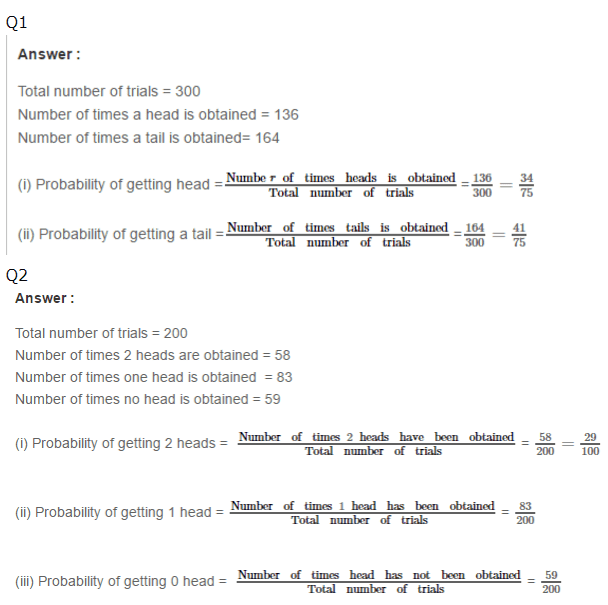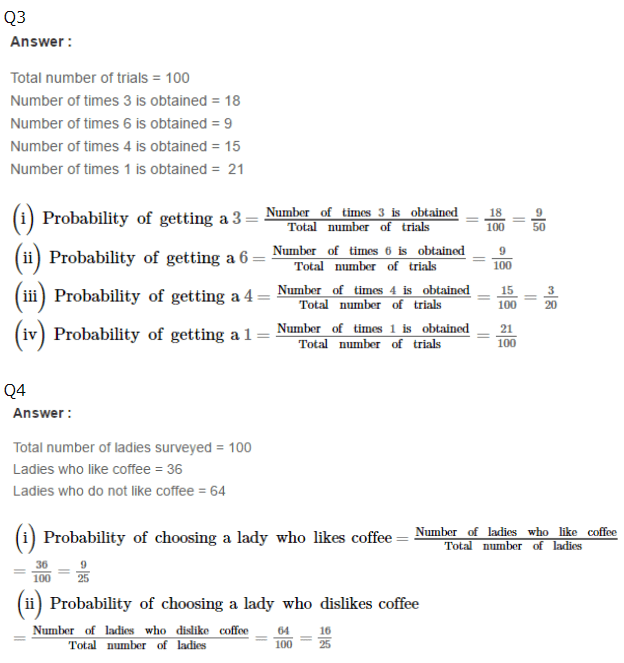RS Aggarwal Class 7 Solutions Chapter 23 - Probability

RS Aggarwal Class 7 Chapter 23 - Probability Solutions Free PDF

The extent to which something is likely to occur is called Probability and it always lies between 0 and 1, where 0 indicates impossibility and 1 dictates the probability. The lower the probability of an event occurring, means the less likely the event will actually happen. It can be either represented in a fraction form or a decimal form.

Probability has a wide range of applications in many different fields, some of these areas are:

1. Statistics
2. Mathematics
3. Game Theory
4. Computer Science
5. Philosophy
6. Science
7. Artificial Intelligence
8. Gambling
9. Philosophy

Get the RS Aggarwal Class 7 Solutions Chapter 23 Probability below:Practise This Question

The compound which has only primary and tertiary carbons is?